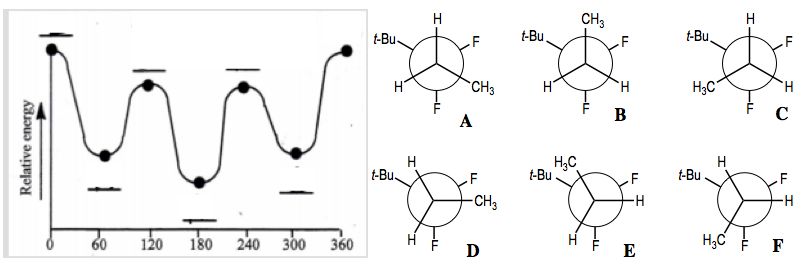# Problem: Write the letter corresponding to the correct conformer that represents the relative potential energy of the minima and maxima dots as the Newman projection's dihedral angle rotates 360 degrees.

🤓 Based on our data, we think this question is relevant for Professor Raker's class at USF.

###### Problem Details

Write the letter corresponding to the correct conformer that represents the relative potential energy of the minima and maxima dots as the Newman projection's dihedral angle rotates 360 degrees.What scientific concept do you need to know in order to solve this problem?

Our tutors have indicated that to solve this problem you will need to apply the Dihedral Angle concept. If you need more Dihedral Angle practice, you can also practice Dihedral Angle practice problems.

What is the difficulty of this problem?

Our tutors rated the difficulty ofWrite the letter corresponding to the correct conformer that...as medium difficulty.

How long does this problem take to solve?

Our expert Organic tutor, Jonathan took 4 minutes and 7 seconds to solve this problem. You can follow their steps in the video explanation above.

What professor is this problem relevant for?

Based on our data, we think this problem is relevant for Professor Raker's class at USF.